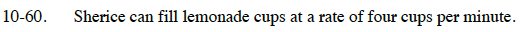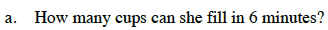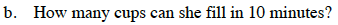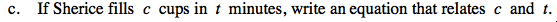### Home > CC3 > Chapter Ch10 > Lesson 10.1.4 > Problem10-60

10-60.
1. Sherice can fill lemonade cups at a rate of four cups per minute. Homework Help ✎

1. How many cups can she fill in 6 minutes?

2. How many cups can she fill in 10 minutes?

3. If Sherice fills c cups in t minutes, write an equation that relates c and t.To find the number of cups filled in a certain amount of time, multiply the rate by the time.

$\left(\frac{4\; \text{cups}}{\text{minute}}\right)\cdot (6\; \text{minutes})$

24 cupsSee the help for part (a).Notice that in parts (a) and (b), the rate must be multiplied by time (t) in order to find the number of cups (c).

c = 4t